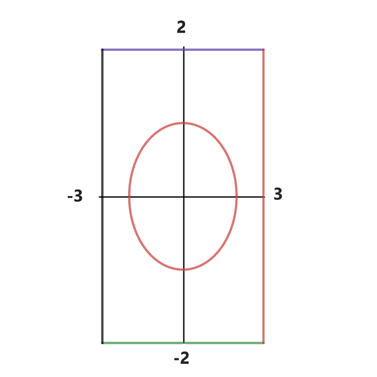# To graph : The quadratic surface x 2 4 + y 2 = 1 in an appropriate viewing rectangle.### Precalculus: Mathematics for Calcu...

6th Edition
Stewart + 5 others
Publisher: Cengage Learning
ISBN: 9780840068071### Precalculus: Mathematics for Calcu...

6th Edition
Stewart + 5 others
Publisher: Cengage Learning
ISBN: 9780840068071

#### Solutions

Chapter 1, Problem 122RE
To determine

## To graph: The quadratic surface x24+y2=1 in an appropriate viewing rectangle.

Expert Solution

### Explanation of Solution

Given information:

The equation of quadratic surface x24+y2=1

Graph:

The graph of the quadratic surface x24+y2=1 can be sketched by plotting points with the aid of a graphing device.

A viewing rectangle is that rectangular portion of the graph of the given equation in display which depicts the viewing window in which the graph van be fitted suitably. The need for viewing rectangle arose because the default screen does not display the complete information at times, which can be misleading too.

Therefore, the viewing rectangle must be chosen with care.

In general, if the x -values and y -values are chosen in such a way that they lie between:

Xmin=aXmax=bYmin=cYmax=d

Then, it is said that the displayed portion for the given graph lies in the rectangle:

[a,b]×[c,d]={(x,y)|axb,cxd}

Therefore the viewing rectangle is given as [a,b] by [c,d]

Now for the given quadratic surface x24+y2=1 , chose:

Xmin=3Xmax=3Ymin=2Ymax=2

Hence the viewing rectangle is [3,3] by [2,2] and can be seen as follows:Interpretation:

Now for the given quadratic surface x24+y2=1 , it can be seen that:

Xmin=3Xmax=3Ymin=2Ymax=2

Hence the viewing rectangle is [3,3] by [2,2] is the appropriate representation of the graph.

Moreover if a viewing rectangle with smaller dimensions are chosen then the graph fails to fit in it, thereby giving the incomplete or misleading information about the quadratic equation.

Similarly if a viewing rectangle with much larger dimensions are chosen then the graph depicts a lot of extra and unnecessary information which is not required.

Thus, this is the most appropriate representation for the graph.

### Have a homework question?

Subscribe to bartleby learn! Ask subject matter experts 30 homework questions each month. Plus, you’ll have access to millions of step-by-step textbook answers!